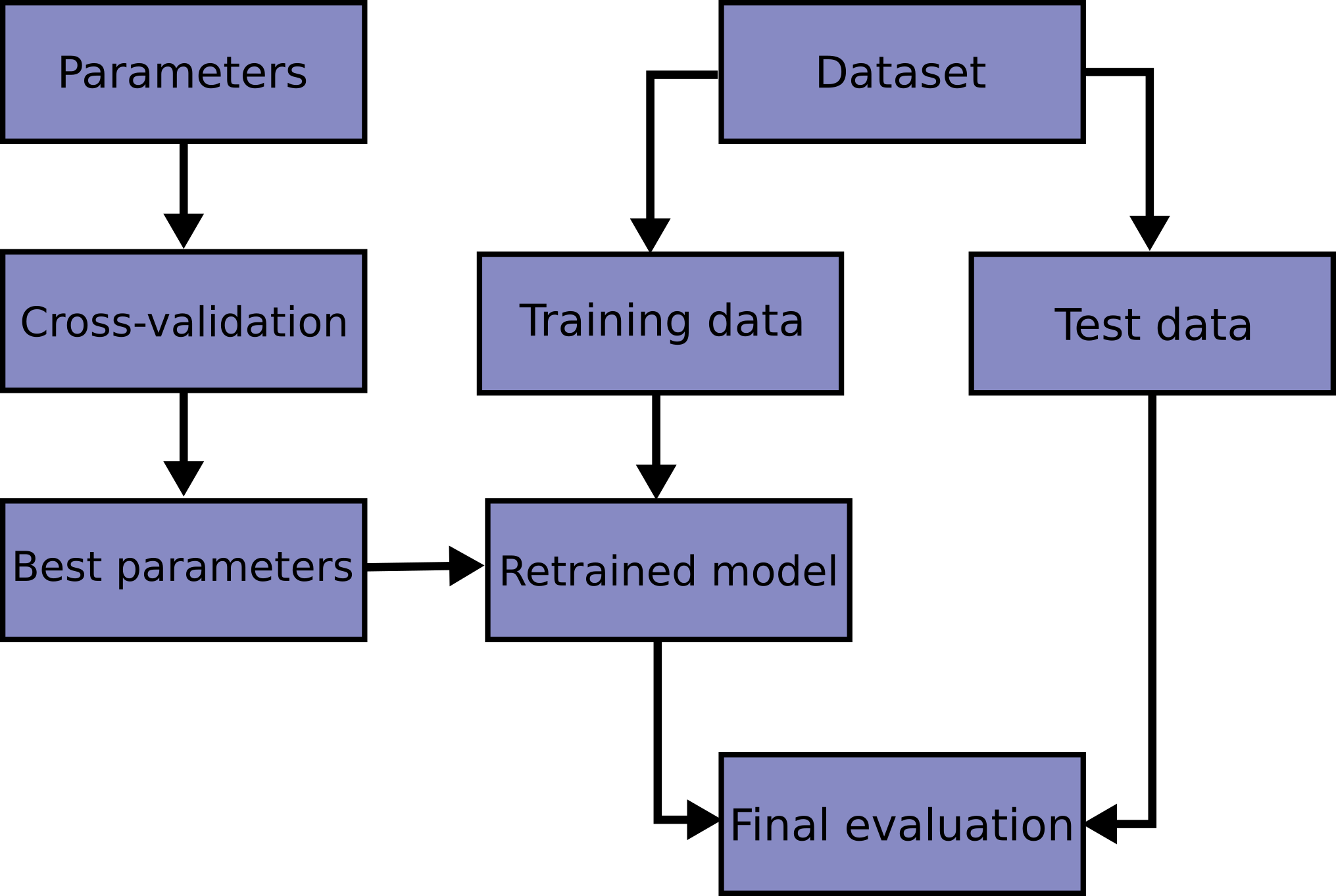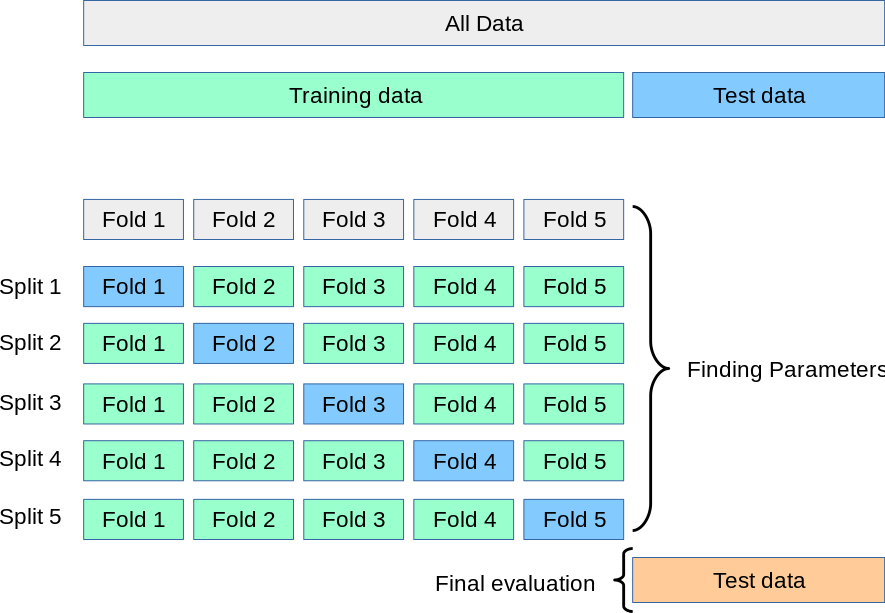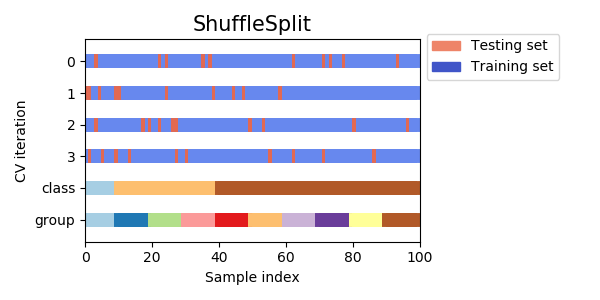# 3.1. 交叉验证：评估估算器的表现``````>>> import numpy as np
>>> from sklearn.model_selection import train_test_split
>>> from sklearn import datasets
>>> from sklearn import svm

>>> iris.data.shape, iris.target.shape
((150, 4), (150,))

``````

``````>>> X_train, X_test, y_train, y_test = train_test_split(
...     iris.data, iris.target, test_size=0.4, random_state=0)

>>> X_train.shape, y_train.shape
((90, 4), (90,))
>>> X_test.shape, y_test.shape
((60, 4), (60,))

>>> clf = svm.SVC(kernel='linear', C=1).fit(X_train, y_train)
>>> clf.score(X_test, y_test)
0.96...

``````

•份训练集子集作为 training data （训练集）训练模型，
• 将剩余的 1 份训练集子集用于模型验证（也就是把它当做一个测试集来计算模型的性能指标，例如准确率）。

k-折交叉验证得出的性能指标是循环计算中每个值的平均值。 该方法虽然计算代价很高，但是它不会浪费太多的数据（如固定任意测试集的情况一样）， 在处理样本数据集较少的问题（例如，逆向推理）时比较有优势。## 3.1.1. 计算交叉验证的指标

``````>>> from sklearn.model_selection import cross_val_score
>>> clf = svm.SVC(kernel='linear', C=1)
>>> scores = cross_val_score(clf, iris.data, iris.target, cv=5)
>>> scores
array([0.96..., 1.  ..., 0.96..., 0.96..., 1.        ])
``````

``````>>> print("Accuracy: %0.2f (+/- %0.2f)" % (scores.mean(), scores.std() * 2))
Accuracy: 0.98 (+/- 0.03)

``````

``````>>> from sklearn import metrics
>>> scores = cross_val_score(
...     clf, iris.data, iris.target, cv=5, scoring='f1_macro')
>>> scores
array([0.96..., 1.  ..., 0.96..., 0.96..., 1.        ])

``````

`cv` 参数是一个整数时， `cross_val_score` 默认使用 `KFold``StratifiedKFold` 策略，后者会在估计器派生自 `ClassifierMixin` 时使用。

``````>>> from sklearn.model_selection import ShuffleSplit
>>> n_samples = iris.data.shape
>>> cv = ShuffleSplit(n_splits=5, test_size=0.3, random_state=0)
>>> cross_val_score(clf, iris.data, iris.target, cv=cv)
array([0.977..., 0.977..., 1.  ..., 0.955..., 1.        ])
``````

``````>>> def custom_cv_2folds(X):
...     n = X.shape
...     i = 1
...     while i <= 2:
...         idx = np.arange(n * (i - 1) / 2, n * i / 2, dtype=int)
...         yield idx, idx
...         i += 1
...
>>> custom_cv = custom_cv_2folds(iris.data)
>>> cross_val_score(clf, iris.data, iris.target, cv=custom_cv)
array([1.        , 0.973...])
``````

from sklearn import preprocessing X_train, X_test, y_train, y_test = train_test_split( ... iris.data, iris.target, test_size=0.4, random_state=0) scaler = preprocessing.StandardScaler().fit(X_train) X_train_transformed = scaler.transform(X_train) clf = svm.SVC(C=1).fit(X_train_transformed, y_train) X_test_transformed = scaler.transform(X_test) clf.score(X_test_transformed, y_test)
0.9333... `[`Pipeline`](https://scikit-learn.org/stable/modules/generated/sklearn.pipeline.Pipeline.html#sklearn.pipeline.Pipeline "sklearn.pipeline.Pipeline") 可以更容易地组合估计器，在交叉验证下使用如下:`py from sklearn.pipeline import make_pipeline clf = make_pipeline(preprocessing.StandardScaler(), svm.SVC(C=1)) cross_val_score(clf, iris.data, iris.target, cv=cv) ...
array([ 0.97..., 0.93..., 0.95...]) ``` 可以参阅 Pipeline（管道）和 FeatureUnion（特征联合）: 合并的评估器.

### 3.1.1.1. cross_validate 函数和多度量评估

`cross_validate` 函数与 `cross_val_score` 在下面的两个方面有些不同 -

• 它允许指定多个指标进行评估.
• 除了测试得分之外，它还会返回一个包含训练得分，拟合次数， score-times （得分次数）的一个字典。 It returns a dict containing training scores, fit-times and score-times in addition to the test score.

`return_train_score` 默认设置为 `True` 。 它增加了所有 scorers(得分器) 的训练得分 keys 。如果不需要训练 scores ，则应将其明确设置为 `False`

``````>>> from sklearn.model_selection import cross_validate
>>> from sklearn.metrics import recall_score
>>> scoring = ['precision_macro', 'recall_macro']
>>> clf = svm.SVC(kernel='linear', C=1, random_state=0)
>>> scores = cross_validate(clf, iris.data, iris.target, scoring=scoring,
...                         cv=5)
>>> sorted(scores.keys())
['fit_time', 'score_time', 'test_precision_macro', 'test_recall_macro']
>>> scores['test_recall_macro']
array([0.96..., 1.  ..., 0.96..., 0.96..., 1.        ])

``````

``````>>> from sklearn.metrics.scorer import make_scorer
>>> scoring = {'prec_macro': 'precision_macro',
...            'rec_macro': make_scorer(recall_score, average='macro')}
>>> scores = cross_validate(clf, iris.data, iris.target, scoring=scoring,
...                         cv=5, return_train_score=True)
>>> sorted(scores.keys())
['fit_time', 'score_time', 'test_prec_macro', 'test_rec_macro',
'train_prec_macro', 'train_rec_macro']
>>> scores['train_rec_macro']
array([0.97..., 0.97..., 0.99..., 0.98..., 0.98...])
``````

``````>>> scores = cross_validate(clf, iris.data, iris.target,
...                         scoring='precision_macro', cv=5,
...                         return_estimator=True)
>>> sorted(scores.keys())
['estimator', 'fit_time', 'score_time', 'test_score']
``````

### 3.1.1.2. 通过交叉验证获取预测

`cross_val_predict`函数的结果可能会与`cross_val_score`函数的结果不一样，因为在这两种方法中元素的分组方式不一样。函数`cross_val_score`在所有交叉验证的折子上取平均。但是，函数`cross_val_predict`只是简单的返回由若干不同模型预测出的标签或概率。因此,`cross_val_predict`不是一种适当的泛化错误的度量。

## 3.1.2. 交叉验证迭代器

### 3.1.2.1. 交叉验证迭代器–循环遍历数据

#### 3.1.2.1.1. K 折

`KFold` 将所有的样例划分为个组，称为折叠 (fold) （如果， 这等价于 Leave One Out（留一） 策略），都具有相同的大小（如果可能）。预测函数学习时使用个折叠中的数据，最后一个剩下的折叠会用于测试。

``````>>> import numpy as np
>>> from sklearn.model_selection import KFold

>>> X = ["a", "b", "c", "d"]
>>> kf = KFold(n_splits=2)
>>> for train, test in kf.split(X):
...     print("%s  %s" % (train, test))
[2 3] [0 1]
[0 1] [2 3]

``````

``````>>> X = np.array([[0., 0.], [1., 1.], [-1., -1.], [2., 2.]])
>>> y = np.array([0, 1, 0, 1])
>>> X_train, X_test, y_train, y_test = X[train], X[test], y[train], y[test]

``````

#### 3.1.2.1.2. 重复 K-折交叉验证

`RepeatedKFold` 重复 K-Fold n 次。当需要运行时可以使用它 `KFold` n 次，在每次重复中产生不同的分割。

2折 K-Fold 重复 2 次的示例:

``````>>> import numpy as np
>>> from sklearn.model_selection import RepeatedKFold
>>> X = np.array([[1, 2], [3, 4], [1, 2], [3, 4]])
>>> random_state = 12883823
>>> rkf = RepeatedKFold(n_splits=2, n_repeats=2, random_state=random_state)
>>> for train, test in rkf.split(X):
...     print("%s  %s" % (train, test))
...
[2 3] [0 1]
[0 1] [2 3]
[0 2] [1 3]
[1 3] [0 2]

``````

#### 3.1.2.1.3. 留一交叉验证 (LOO)

`LeaveOneOut` (或 LOO) 是一个简单的交叉验证。每个学习集都是通过除了一个样本以外的所有样本创建的，测试集是被留下的样本。 因此，对于个样本，我们有个不同的训练集和个不同的测试集。这种交叉验证程序不会浪费太多数据，因为只有一个样本是从训练集中删除掉的:

``````>>> from sklearn.model_selection import LeaveOneOut

>>> X = [1, 2, 3, 4]
>>> loo = LeaveOneOut()
>>> for train, test in loo.split(X):
...     print("%s  %s" % (train, test))
[1 2 3] 
[0 2 3] 
[0 1 3] 
[0 1 2] 

``````

LOO 潜在的用户选择模型应该权衡一些已知的警告。 当与折交叉验证进行比较时，可以从样本中构建模型，而不是模型，其中。 此外，每个在个样本而不是在上进行训练。在两种方式中，假设不是太大，并且， LOO 比折交叉验证计算开销更加昂贵。

#### 3.1.2.1.4. 留 P 交叉验证 (LPO)

`LeavePOut``LeaveOneOut` 非常相似，因为它通过从整个集合中删除个样本来创建所有可能的 训练/测试集。对于个样本，这产生了个 训练-测试 对。与 `LeaveOneOut``KFold` 不同，当时，测试集会重叠。

``````>>> from sklearn.model_selection import LeavePOut

>>> X = np.ones(4)
>>> lpo = LeavePOut(p=2)
>>> for train, test in lpo.split(X):
...     print("%s  %s" % (train, test))
[2 3] [0 1]
[1 3] [0 2]
[1 2] [0 3]
[0 3] [1 2]
[0 2] [1 3]
[0 1] [2 3]

``````

#### 3.1.2.1.5. 随机排列交叉验证 a.k.a. Shuffle & Split

`ShuffleSplit`

`ShuffleSplit` 迭代器 将会生成一个用户给定数量的独立的训练/测试数据划分。样例首先被打散然后划分为一对训练测试集合。

``````>>> from sklearn.model_selection import ShuffleSplit
>>> X = np.arange(5)
>>> ss = ShuffleSplit(n_splits=3, test_size=0.25,
...     random_state=0)
>>> for train_index, test_index in ss.split(X):
...     print("%s  %s" % (train_index, test_index))
...
[1 3 4] [2 0]
[1 4 3] [0 2]
[4 0 2] [1 3]

```````ShuffleSplit` 可以替代 `KFold` 交叉验证，因为其提供了细致的训练 / 测试划分的 数量和样例所占的比例等的控制。

### 3.1.2.2. 基于类标签、具有分层的交叉验证迭代器

#### 3.1.2.2.1. 分层 k 折

`StratifiedKFold`k-fold 的变种，会返回 stratified（分层） 的折叠：每个小集合中， 各个类别的样例比例大致和完整数据集中相同。

``````>>> from sklearn.model_selection import StratifiedKFold

>>> X = np.ones(10)
>>> y = [0, 0, 0, 0, 1, 1, 1, 1, 1, 1]
>>> skf = StratifiedKFold(n_splits=3)
>>> for train, test in skf.split(X, y):
...     print("%s  %s" % (train, test))
[2 3 6 7 8 9] [0 1 4 5]
[0 1 3 4 5 8 9] [2 6 7]
[0 1 2 4 5 6 7] [3 8 9]

``````

`RepeatedStratifiedKFold` 可用于在每次重复中用不同的随机化重复分层 K-Fold n 次。

### 3.1.2.2.2. 分层随机 Split

`StratifiedShuffleSplit`ShuffleSplit 的一个变种，会返回直接的划分，比如： 创建一个划分，但是划分中每个类的比例和完整数据集中的相同。

### 3.1.2.3. 用于分组数据的交叉验证迭代器

#### 3.1.2.3.1. 组 k-fold

`GroupKFold` 是 k-fold 的变体，它确保同一个 group 在测试和训练集中都不被表示。 例如，如果数据是从不同的 subjects 获得的，每个 subject 有多个样本，并且如果模型足够灵活以高度人物指定的特征中学习，则可能无法推广到新的 subject 。 `GroupKFold` 可以检测到这种过拟合的情况。 Imagine you have three subjects, each with an associated number from 1 to 3:

``````>>> from sklearn.model_selection import GroupKFold

>>> X = [0.1, 0.2, 2.2, 2.4, 2.3, 4.55, 5.8, 8.8, 9, 10]
>>> y = ["a", "b", "b", "b", "c", "c", "c", "d", "d", "d"]
>>> groups = [1, 1, 1, 2, 2, 2, 3, 3, 3, 3]

>>> gkf = GroupKFold(n_splits=3)
>>> for train, test in gkf.split(X, y, groups=groups):
...     print("%s  %s" % (train, test))
[0 1 2 3 4 5] [6 7 8 9]
[0 1 2 6 7 8 9] [3 4 5]
[3 4 5 6 7 8 9] [0 1 2]

``````

#### 3.1.2.3.2. 留一组交叉验证

`LeaveOneGroupOut` 是一个交叉验证方案，它根据第三方提供的 array of integer groups （整数组的数组）来提供样本。这个组信息可以用来编码任意域特定的预定义交叉验证折叠。

``````>>> from sklearn.model_selection import LeaveOneGroupOut

>>> X = [1, 5, 10, 50, 60, 70, 80]
>>> y = [0, 1, 1, 2, 2, 2, 2]
>>> groups = [1, 1, 2, 2, 3, 3, 3]
>>> logo = LeaveOneGroupOut()
>>> for train, test in logo.split(X, y, groups=groups):
...     print("%s  %s" % (train, test))
[2 3 4 5 6] [0 1]
[0 1 4 5 6] [2 3]
[0 1 2 3] [4 5 6]

``````

#### 3.1.2.3.3. 留 P 组交叉验证

`LeavePGroupsOut` 类似于 `LeaveOneGroupOut` ，但为每个训练/测试集删除与组有关的样本。

Leave-2-Group Out 的示例:

``````>>> from sklearn.model_selection import LeavePGroupsOut

>>> X = np.arange(6)
>>> y = [1, 1, 1, 2, 2, 2]
>>> groups = [1, 1, 2, 2, 3, 3]
>>> lpgo = LeavePGroupsOut(n_groups=2)
>>> for train, test in lpgo.split(X, y, groups=groups):
...     print("%s  %s" % (train, test))
[4 5] [0 1 2 3]
[2 3] [0 1 4 5]
[0 1] [2 3 4 5]

``````

#### 3.1.2.3.4. Group Shuffle Split

`GroupShuffleSplit` 迭代器是 `ShuffleSplit``LeavePGroupsOut` 的组合，它生成一个随机划分分区的序列，其中为每个分组提供了一个组子集。

``````>>> from sklearn.model_selection import GroupShuffleSplit

>>> X = [0.1, 0.2, 2.2, 2.4, 2.3, 4.55, 5.8, 0.001]
>>> y = ["a", "b", "b", "b", "c", "c", "c", "a"]
>>> groups = [1, 1, 2, 2, 3, 3, 4, 4]
>>> gss = GroupShuffleSplit(n_splits=4, test_size=0.5, random_state=0)
>>> for train, test in gss.split(X, y, groups=groups):
...     print("%s  %s" % (train, test))
...
[0 1 2 3] [4 5 6 7]
[2 3 6 7] [0 1 4 5]
[2 3 4 5] [0 1 6 7]
[4 5 6 7] [0 1 2 3]

``````

### 3.1.2.5. 交叉验证在时间序列数据中应用

#### 3.1.2.5.1. 时间序列分割

`TimeSeriesSplit`k-fold 的一个变体，它首先返回折作为训练数据集，并且折作为测试数据集。 请注意，与标准的交叉验证方法不同，连续的训练集是超越前者的超集。 另外，它将所有的剩余数据添加到第一个训练分区，它总是用来训练模型。

``````>>> from sklearn.model_selection import TimeSeriesSplit

>>> X = np.array([[1, 2], [3, 4], [1, 2], [3, 4], [1, 2], [3, 4]])
>>> y = np.array([1, 2, 3, 4, 5, 6])
>>> tscv = TimeSeriesSplit(n_splits=3)
>>> print(tscv)
TimeSeriesSplit(max_train_size=None, n_splits=3)
>>> for train, test in tscv.split(X):
...     print("%s  %s" % (train, test))
[0 1 2] 
[0 1 2 3] 
[0 1 2 3 4] 

``````

## 3.1.3. A note on shuffling

• 这种方式仅需要很少的内存就可以打散数据。
• 默认不会进行打散，包括设置 `cv=some_integer` （直接）k 折叠交叉验证的 `cross_val_score` ， 表格搜索等。注意 `train_test_split` 会返回一个随机的划分。
• 参数 `random_state` 默认设置为 `None` ，这意为着每次进行 `KFold(..., shuffle=True)` 时，打散都是不同的。 然而， `GridSearchCV` 通过调用 `fit` 方法验证时，将会使用相同的打散来训练每一组参数。
• 为了保证结果的可重复性（在相同的平台上），应该给 `random_state` 设定一个固定的值。